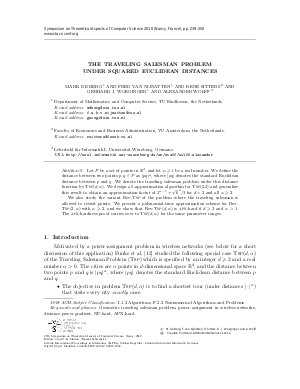Document# The Traveling Salesman Problem under Squared Euclidean Distances

### Authors Fred van Nijnatten, René Sitters, Gerhard J. Woeginger, Alexander Wolff, Mark de Berg## File

LIPIcs.STACS.2010.2458.pdf
• Filesize: 329 kB
• 12 pages

## Cite As

Fred van Nijnatten, René Sitters, Gerhard J. Woeginger, Alexander Wolff, and Mark de Berg. The Traveling Salesman Problem under Squared Euclidean Distances. In 27th International Symposium on Theoretical Aspects of Computer Science. Leibniz International Proceedings in Informatics (LIPIcs), Volume 5, pp. 239-250, Schloss Dagstuhl - Leibniz-Zentrum für Informatik (2010)
https://doi.org/10.4230/LIPIcs.STACS.2010.2458

## Abstract

Let $P$ be a set of points in $\Reals^d$, and let $\alpha \ge 1$ be a real number. We define the distance between two points $p,q\in P$ as $|pq|^{\alpha}$, where $|pq|$ denotes the standard Euclidean distance between $p$ and $q$. We denote the traveling salesman problem under this distance function by \tsp($d,\alpha$). We design a 5-approximation algorithm for \tsp(2,2) and generalize this result to obtain an approximation factor of $3^{\alpha-1}+\sqrt{6}^{\,\alpha}\!/3$ for $d=2$ and all $\alpha\ge2$. We also study the variant Rev-\tsp\ of the problem where the traveling salesman is allowed to revisit points. We present a polynomial-time approximation scheme for Rev-\tsp$(2,\alpha)$ with $\alpha\ge2$, and we show that Rev-\tsp$(d, \alpha)$ is \apx-hard if $d\ge3$ and $\alpha>1$. The \apx-hardness proof carries over to \tsp$(d, \alpha)$ for the same parameter ranges.
##### Keywords
• Geometric traveling salesman problem
• power-assignment in wireless networks
• NP-hard
• APX-hard

## Metrics

• Access Statistics
• Total Accesses (updated on a weekly basis)
0